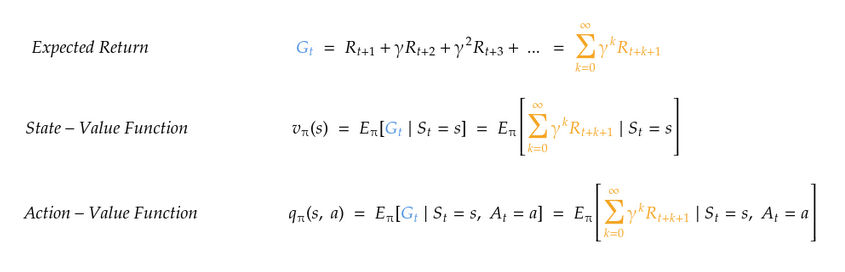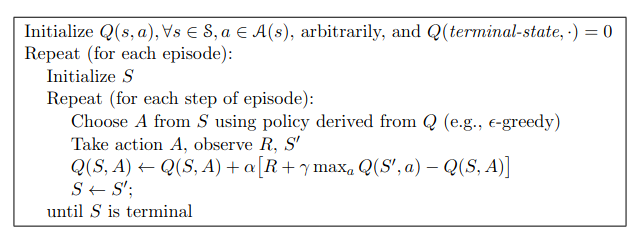# Understand the Q-Learning algorithm step by step, as well as the main components of any RL-based systemJavier Martinez

5 months ago | 7 min read

We have all experienced a situation in which we have done something wrong, been punished for it, and this has made us not to do it again. In the same way, many times good actions are rewarded, encouraging us to repeat them on more occasions. Following this parallelism, Reinforcement Learning agents will take certain actions following a strategy/policy, and receive positive or negative feedback, depending on whether the action taken is beneficial or not. This reward is then used to update the policy, and the whole process is repeated until an optimal policy is reached, as shown in Figure 1.

In psychology, there is a learning procedure called Instrumental or Operant Conditioning , which is based on the fact that the probability that a given behavior will occur depends on the expected consequences. The main goal of this discipline is to increase or decrease the probability that a behavior will be repeated, which is exactly the same approach as that of Reinforced Learning!

The objective of the Reinforced Learning agent is to optimize the actions taken in order to obtain the highest possible rewards, through successive interactions of the agent with a dynamic environment.

It can be seen, therefore, how the main premise of Reinforced Learning is based on biological learning procedures, and how these procedures have been shown to be effective throughout human history, which makes it one of the most exciting and interesting branches of Machine Learning.

## Model-Free and Model-Based RL algorithms

Before proceeding with the explanation and implementation of the Q-learning algorithm, it is important to note that RL algorithms are divided into two main groups: Model-Based algorithms and Model-Free algorithms.

The former aim to learn a model of the environment through interactions with it, such that the agent is able to predict the reward of a given action before taking it (by having a model of the environment, it can foresee what will happen after each action), thus allowing for action planning. On the other hand, model-free algorithms must take an action in order to observe its consequences, and then earn from it (see Figure 2). It should be noted that the word ‘model’ does not refer to Machine Learning models, but to a model of the environment itself. For a more in-depth explanation of the differences between these two groups, take a look at this article .

As will be seen later, Q-Learning is a Model-Free algorithm, since its learning consists of taking actions, receiving the reward, and learning from the consequence of taking those actions.

## Q-Learning

The Q-learning algorithm makes use of a Q-table (2D matrix) containing state-action pairs, such that each value in the table/matrix, Q(S, A), corresponds to the Q-value estimate of taking action S in state A (Q-Values will be introduced later). As the agent interacts with the environment, the Q-values of the Q-table will converge to their optimal values, until the optimal policy is found.

It is complicated at first to understand all these terms, and it is normal that many questions arise: What is a Q-Value? How is a Q-Table constructed? How are the Q-values updated?

All the concepts necessary to understand the flow of the algorithm, as well as the questions above, are explained below.

## Q-Table Construction

As previously mentioned, the Q-table is a matrix where each element corresponds to a state-action pair. Therefore, the Q-table will be a mxn matrix, where m is the number of possible states, and n the number of possible actions. The Q-values of the Q-table must have an initial value, so the table will be initialized with all its values set to zero.

Example:

In order to make it simple, the environment will be a room with 4 possible states (A, B, C, D), as shown in the image. In addition, the agent will be able to perform 4 possible actions: go up, down, left and right.

Taking into account the agent and environment mentioned above, the Q-table will be a 4x4 matrix, with 4 rows corresponding to the 4 possible states, and 4 columns corresponding to the 4 possible actions. As can be seen below, all values have been initialized to zero.

## Q-Values

Once the Q-table is initialized, the agent can start interacting with the environment, and updating the Q-values to achieve the optimal policy. But, how are the Q-values updated?

First of all, it is important to introduce the concept of Value Function, which appeared earlier in Figure 2. The Value Function is a measure of how beneficial it is for an agent to be in a given state, or in a given state-action pair.

There are two types of Value Functions: State-Value Function, v(S), which determines the benefit of being in a particular state while following a certain policy; and Action-Value Function, q(S, A), which determines the benefit of taking a particular action from a particular state while following a certain policy. To be more concrete, these functions return the expected benefit of starting in a state (for State-Value Function) or a state-action pair (for Action-Value Function), and following the given policy.

Both functions are shown below, as well as a link to an article that explains in depth their importance and raison d’être.Equations for Expected Return, State-Value and Action-Value Functions. Image by author

The result of the Action-Value Function is known as Q-value, which, as mentioned before, is each of the cells that make up the Q-table. Therefore, the Q-table is providing us with the expected benefits of taking a certain action from a certain state, information that the agent will use to move optimally through the environment. Because of this, the goal of the agent will be to find the optimal Action-Value Function, q*(S, A), (optimal Q-values), such that it returns the highest possible return from any state-action pair, following any policy.

## Q-Values Update

A property of the optimal Action-Value Function, q*(S, A), is that it satisfies Bellman’s optimality equation, shown below. Knowing this, Bellman’s equation can be used to infer the optimal Action-Value Function iteratively, which is the main goal of the agent.

In the case of Q-Learning, an adaptation of Bellman’s optimality equation shown in Figure 3 is used to iteratively update the Q-values of the Q-table. This equation is used to reduce the error by comparing the current Q-value with the optimum one in each iteration, seeking to equalize both.

Note that the Q-values update equation makes use of an α parameter, called learning rate, which indicates how heavily the new Q-value will be weighted in each update/iteration. A learning rate of 0 will cause the agent to never update its Action-Value Function, while a learning rate of 1 will cause only the new learned Q-value to be considered. It is common to find the ideal value of this parameter by trial and error.

## Algorithm Flow

Now that all the components and steps of the algorithm are explained, it is time to put it all together and make the agent learn. Below is the pseudocode of the algorithm, which will be used as a reference during the implementation of Q-Learning.Q-Learning pseudocode. Extracted from Sutton and Barto: “Reinforcement Learning: An Introduction” 

1. Initialize Q-Table

The Q-Table is initialized with shape dependent on the number of possible states and actions, and all its values set to zero, as explained before.

2. Start Episode

Each episode will run until the agent reaches a terminal/goal state. The agent starts the episode from a random state, and for each timestep within an episode it will:

1) Take an action following a policy (the most commonly used for this algorithm is ɛ-greedy policy).

The ɛ-greedy policy tries to strike a balance between randomly exploring the environment in order to learn, and exploiting it by selecting the optimal actions according to the Q-values. The tendency of the policy towards exploration or exploitation of the environment is given by the epsilon ɛ parameter, allowing the policy to start with an exploratory behavior and dynamically changing it to an exploitative one. For more information about this policy, see .

2) Calculate the new Q-value from the new state reached and reward obtained, following the Q-Value Updation equation mentioned previously.

3) Start the next timestep from the new state reached.

Training will end when all episodes have been completed. At this point, the Q-values of the Q-table will be optimal (as long as the training worked), which means that the agent will obtain the maximum returns in each state S if he chooses the action A with the highest Q-value.

Finally, in order to use the trained agent in a non-training environment, it will only be necessary to make it choose the action with the highest Q-Value in each timestep, since the Q-Table has already been optimised during training.

## Code

The complete implementation of the Q-Learning algorithm, together with visualizations and evaluation of the trained agent, can be found in my GitHub repository as a Jupyter Notebook, in order to facilitate understanding and learning.

Github Repository

I have to mention, however, that this article only refers to the theoretical and introductory concepts of the algorithm, and does not focus on its implementation in code. This is because this month I will publish another article, exclusively focused on the implementation of the algorithm and its practical application to a known OpenAI Gym environment. Follow me if you are interested so you don’t miss out!

## Original Article

This article has been previously published on my Medium account, through the publication Towards Data Science. You can access the original article from the following link:

Applied Reinforcement Learning I: Q-Learning

## References

 STADDON, John ER; CERUTTI, Daniel T. Operant conditioning. Annual review of psychology, 2003, vol. 54, p. 115

 Model-Based and Model-Free Reinforcement Learninghttps://neptune.ai/blog/model-based-and-model-free-reinforcement-learning-pytennis-case-study

 SUTTON, Richard S.; BARTO, Andrew G. Reinforcement learning: An introduction. MIT press, 2018

 Epsilon-Greedy Algorithm in Reinforcement Learning https://www.geeksforgeeks.org/epsilon-greedy-algorithm-in-reinforcement-learning/

UpvoteCreated by

Javier Martinez

Artificial Intelligence | Robotics | Industrial EngineeringPost

Upvote

Downvote

Comment

Bookmark

Share

Related Articles